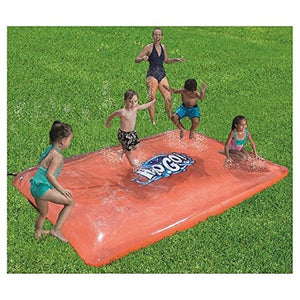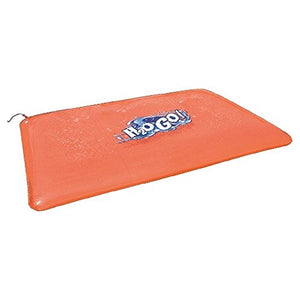# H2Ogo Splash Blobz Splat Mat Pad

Vendor
Bestway
Regular price
\$116.28
Sale price
\$82.62
Quantity must be 1 or more

• 9

• .

• 8

• F

• T

• X

• 6

• .

• 6

• F

• T

• S

• l

• i

• d

• e

• ,

• J

• u

• m

• p

• a

• n

• d

• S

• p

• l

• a

• s

• h

• W

• a

• t

• e

• r

• F

• i

• l

• l

• e

• d

• G

• i

• a

• n

• t

• S

• p

• r

• a

• y

• i

• n

• g

• S

• p

• l

• a

• s

• h

• M

• a

• t

• !

• H

• o

• s

• e

• A

• t

• t

• a

• c

• h

• m

• e

• n

• t

• B

• u

• i

• l

• t

• -

• I

• n

• S

• p

• r

• i

• n

• k

• l

• e

• r

• A

• g

• e

• s

• 3

• +

• D

• e

• s

• i

• g

• n

• e

• d

• W

• a

• t

• e

• r

• C

• a

• p

• a

• c

• i

• t

• y

• 2

• 3

• 9

• g

• a

• l

• .

• (

• 9

• 0

• 6

• L

• )

9.8FT X 6.6 FT Slide, Jump and Splash Water Filled Giant Spraying Splash Mat! Hose Attachment Built-In Sprinkler Ages 3 + Designed Water Capacity 239 gal. (906L)